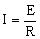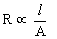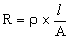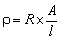# Chemistry Assignment Help with Conductance of Electrolytes## 9.3 Conductance of Electrolytes

We have seen that electrolytes solutions conduct electrical currents through them by movement of the ions to the electrodes. The power of electrolytes to conduct electrical currents is termed conductivity or conductance. Like metallic conductors, electrolytes obey Ohm’s law. According to this law, the current I flowing through a metallic conductor is given by the relationWhere E is the potential difference at two ends (in volts); and R is the resistance measured in ohms (W). The resistance R of a conductor is directly proportional to its length, l, and inversely proportional to the area of its cross-section, A. That is,orWhere r ‘‘rho’’ is a constant of proportionality and is called resistivity or specific resistance. Its value depends upon the material of the conductor.If l = 1 cm and A = 1 sq cm, then

r = R

Thus it follows that the Specific resistance of a conductor is the resistance in ohms which one centimeter cube of it offers to the passage of electricity.

### Email Based Assignment Help in Conductance of Electrolytes

We are the leading online Assignment Help provider. Find answers to all of your doubts regarding the Conductance of Electrolytes in chemistry. We at assignmenthelp.net provide homework, Assignment Help to the school, college or university level students. Our expert online tutors are available to help you in Conductance of Electrolytes. Our service is focused on: time delivery, superior quality, creativity and originality.